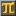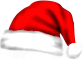#SCIENTIFIC CALCULATORThis calculation: Edit - Share - Print -
Save

• Press Ctrl-d to save this calculation as a bookmark.

- Reset

 Solving 2 linear equations using Cramer's rule. 3x - 7y = 5 5x + 3y = 11 3 * 3 - ( - 7) * 5 =   44.0 [DetA] 5 * 3 - ( - 7) * 11 =   92.0 [DetAx] 3 * 11 - 5 * 5 =   8.0 [DetAy] x: [DetAx] / [DetA] =   2.09090909091 … [x] y: [DetAy] / [DetA] =   0.181818181818 … [y] verification: 3 * [x] - 7 * [y] =   5.0  5 * [x] + 3 * [y] =   11.0  ok
CalculatorPi.comⒸ Troymius LLC